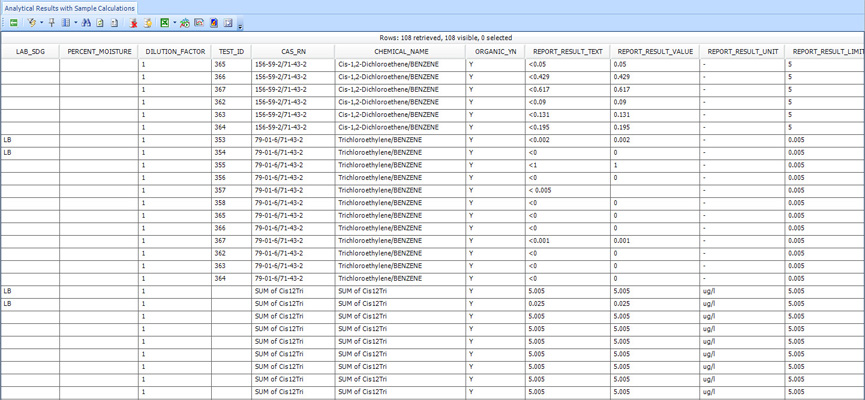﻿ Analytical Results with Sample Calculations

# Analytical Results with Sample Calculations

Report Name: Analytical Results with Sample Calculations (Table) (11472)

Report Type: Standard

Description: The Analytical Results with Sample Calculations (Table) Report generates the results of the Analytical Results, and the results from the calculations of balance and summation of the results of the Analytical Results.

Balance Calculation

The report creates a new record for each sample where the REPORT_RESULT_VALUE (also REPORT_RESULT_TEXT and REPORT_RESULT_LIMIT) equals the original REPORT_RESULT_VALUE divided by the REPORT_RESULT_VALUE of the balance analyte from the same sample, which is defined by FACILITY_ID and SAMPLE_ID. No new rows are added if no balance value is entered or not found in the result.

Sum Calculation

The report creates a new record for each sample where the REPORT_RESULT_VALUE (also REPORT_RESULT_TEXT and REPORT_RESULT_LIMIT)

equals the sum of the results of the analytes for the same sample defined by FACILITY_ID and SAMPLE_ID, where the analytes are selected over

user interface by either individual or groups. No new rows are added if the analytes to be summed are not selected.

 Notes: •It is suggested that RT_MTH_ANL_GROUP_MEMBER.REPORTING_UNITS be used for the result analyte selection of beta AR so that all the units of the results are the same for the purpose of doing the calculations of balance and summation.•If  RT_MTH_ANL_GROUP_MEMBER.REPORTING_UNITS is not used, the report does the unit conversions below. A) The units of all analytes are unified to the unit of balance analyte if balance calculation is carried out. B) The units of all the analytes to be summed are unified to the unit that is the latest (by SAMPLE_DATE) of one summed analyte. If multiple analyte groups are selected, the latest one unit is defined from the all selected analyte groups.•If both By Analyte and By Analyte Group of Sample Summary are selected, the latter is ignored.•All the rows with unit conversion errors or REPORT_RESULT_VALUE=99999999 are removed from the final report results.•All other columns except for REPORT_RESULT_VALUE, REPORT_RESULT_TEXT and REPORT_RESULT_LIMIT are populated based on the attached Analytical Results_Calculations Report Development.xls (Analytical Results_Calculations Report Development.xls).

Un-ionized Ammonia Calculation

The report can compute the Ammonia (Un-ionized Ammonia) based on values of Total Ammonia, Temp C, pH and TDS. Note the Ammonia_union below is the analyte group name for Un-ionized Ammonia as shown in the image below. The report result value of Ammonia is calculated by the equations below.Calculation Equations:

Assume p1-p4 are values of  Total Ammonia, Temp C, pH and TDS

H = 0.09018 + 2729.92 / (p2 + 273.2)

L = 0.000025 * p4

M = 87.74 - 0.4008 * p2 + 0.0009398 * p2 ^ 2 - 0.00000141 * p2 ^ 3

N = 1824830 * (M * (p2 + 273.16)) ^ -1.5

O = -(L * N) ^ 0.5 / (1 + L ^ 0.5)

P = (1 / (1 + 10 ^ (H - p3 - O)))

report_result_value for the Un-ionized Ammonia = Math.Round((p1 * P), decimals)

Report Dependencies: Analytical Results 50943

File Name: EarthSoft.Reports.Library.11472.dll

Contact support@earthsoft.com for a direct file link.

Language: English

Input Parameters: A selection you make to customize the output of a report.

Please note that the Additional Fields parameter may be customized as explained here.

The selections of Analytical Results, plus Sample Computation selections.

Input Parameters

Input Parameter Screenshot

Output Columns

Location

Individual Group(s)

Sample

Type(s)

Date Range

Start

End

Matrix(es)

Delivery Group(s)

Depth

Minimum Start Depth

Maximum End Depth

Unit

Test

Method(s)

Fraction(s)

Column Number(s)

Type(s)

Lab Matrix(es)

Prep Method(s)

Result

Analyte

Individual(s)

Group(s)

Unit

Type(s)

Detect Flag(s)

Non-Detect Multiplier

Non-Detect Symbol

Reportable

Reporting Limit

Sample Computation

Analyte Balance

Sample SummaryFACILITY_ID,

FACILITY_CODE,

SYS_LOC_CODE,

LOC_NAME,

SAMPLE_ID,

SYS_SAMPLE_CODE,

SAMPLE_NAME,

SAMPLE_DATE,

SAMPLE_TYPE_CODE,

START_DEPTH,

END_DEPTH,

DEPTH_UNIT,

MATRIX_CODE,

PARENT_SAMPLE_CODE, ANALYSIS_LOCATION,

LAB_SAMPLE_ID,

LAB_MATRIX_CODE,

LAB_NAME_CODE,

ANALYTIC_METHOD,

ANALYSIS_DATE,

COLUMN_NUMBER,

FRACTION,

TEST_TYPE,

PREP_METHOD,

LEACHATE_METHOD,

LEACHATE_DATE,

LAB_SDG,

PERCENT_MOISTURE,

DILUTION_FACTOR,

TEST_ID,

CAS_RN,

CHEMICAL_NAME,

ORGANIC_YN,

REPORT_RESULT_TEXT, REPORT_RESULT_VALUE, REPORT_RESULT_UNIT, REPORT_RESULT_LIMIT, REPORTABLE_RESULT,

DETECT_FLAG, INTERPRETED_QUALIFIERES, VALIDATOR_QUALIFIERS, LAB_QUALIFIERS,

QUANTITATION_LIMIT, METHOD_DETECTION_LIMIT, REPORTING_DETECTION_LIMIT, DETECTION_LIMIT_UNIT, APPROVAL_CODE,

RESULT_TEXT,

RESULT_NUMERIC,

RESULT_UNIT,

RESULT_TYPE_CODE,

X_COORD,

Y_COORD,

Z_COORD_AVG,

ZFROM,

ZTO,

LONGITUDE,

LATITUDE

Note: * refers to hidden parameters

Tables: DT_SAMPLE/TEST/RESULT,  RT_GROUP, RT_GROUP_MEMBER, RT_MTH_ANL_GROUP

Database Type: SQL

Database Schema: Standard (no custom or add-on schemas required)

Database Version: EQuIS 5.6.2+

Data Requirements: N/A

Report Requirements: N/A

Output Type: PRO Grid

Example Output: Please note that the attached xls file is exported from the PRO Grid.